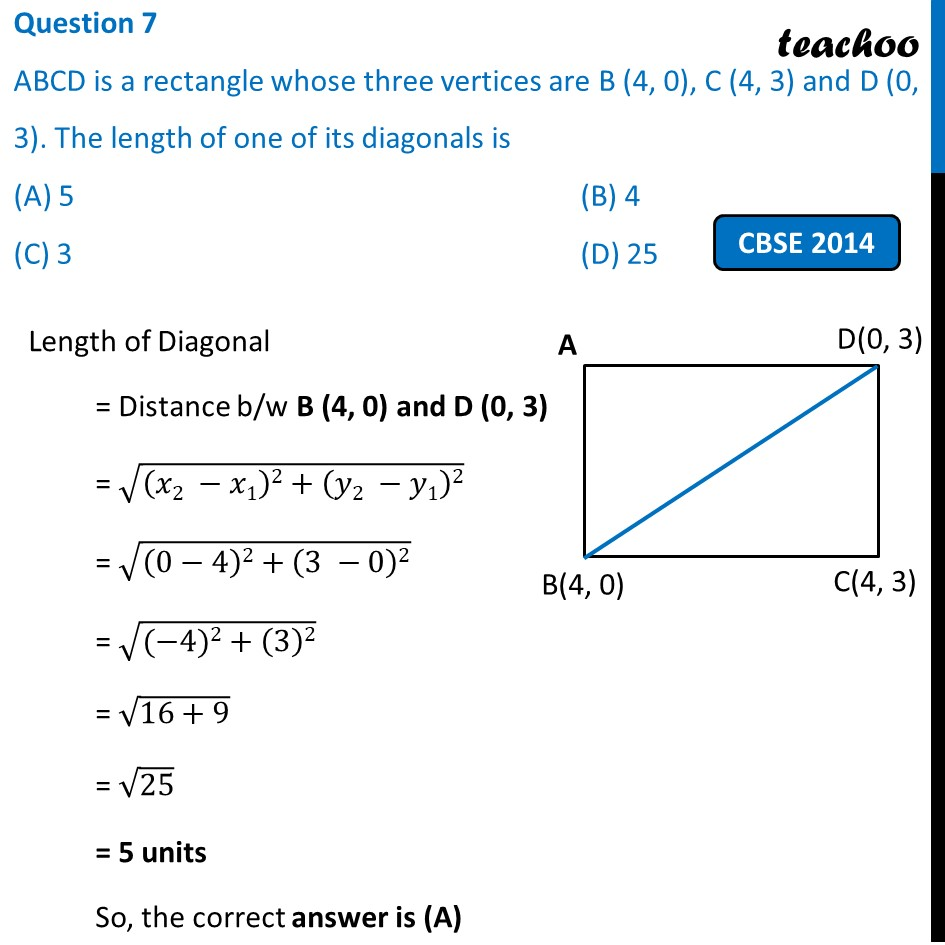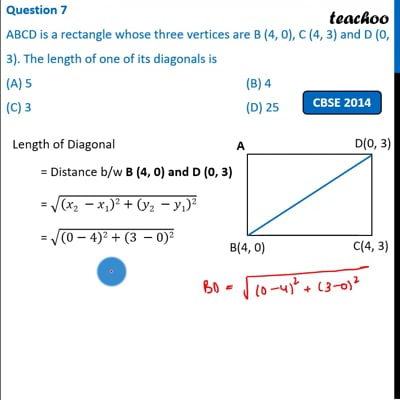Past Year MCQ

Chapter 7 Class 10 Coordinate Geometry
Serial order wise

## (D) 25

This question is simlar to Question 8 - NCERT Exemplar - MCQ - Chapter 7 Class 10 Coordinate GeometryThis video is only available for Teachoo black users

Get live Maths 1-on-1 Classs - Class 6 to 12

### Transcript

Question 7 ABCD is a rectangle whose three vertices are B (4, 0), C (4, 3) and D (0, 3). The length of one of its diagonals is (A) 5 (B) 4 (C) 3 (D) 25 Length of Diagonal = Distance b/w B (4, 0) and D (0, 3) = √((𝑥2 −𝑥1)2+(𝑦2 −𝑦1)2) = √((0−4)2+(3 −0)2) = √((−4)2+(3)2) = √(16+9) = √25 = 5 units So, the correct answer is (A)